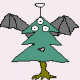# Affine Mess

## January 27, 2009

### Four Points, Two Distances

Filed under: Math Circles — Tags: , — Alon Amit @ 10:53 am

Consider four points in the plane, located at the corners of a square:(The points are shown as slightly large circles just so you can see them better. Imagine those are actual points, with no width or height). The first question we’re going to ask is:

How many distinct distances between pairs of points are there, in this arrangement?

Let’s see what this means, exactly. We have four points. Picking any two of them, we get a cetain distance separating the two. We look at those distances and count how many distinct or different ones there are. So, what do you think? How many?

(a moment of thought…)

Did you get 2? That’s correct. One distance spans an edge of the square, and the other distance is a diagonal of the square. Now this is a fairly special property of arranging four points in this way: there are only 2 distinct distances. So, our challenge question for the day is:

Apart from the corners of a square, can you find other ways of arranging four points in the plane, such that there are only two distinct distances between them?This post is based on a talk I gave at the San Jose Math Circle on 10/1/2008 and at the Bay Area Circle for Teachers. Thanks to Tatiana Shubin, Josh Zucker and Tom Davis for inviting me to participate at the circles.

The images were created using GeoGebra. Try it, it’s great.

1.rhombus with angles 60, 120, 60, 120. 😀

Comment by xx — April 6, 2009 @ 10:35 am

2.That’s right. Can you find other ways? Can you find them all?

(I salute you for commenting without peeking ahead to see the solutions 🙂 )

Comment by Alon Amit — April 6, 2009 @ 11:02 am

3.One more: Equilateral triangle with the forth point at the centroid. Let me think on the others…

Comment by Ori Rosenthal — January 2, 2010 @ 8:04 am

4.i have found this blog, very interesting
i like math problems that come with the answer, it is so much easier 🙂

Comment by erez avraham — January 26, 2010 @ 4:46 pm

5.Thanks for the session today at the math teachers’ circle! http://mathteacherscircle.org

Comment by Joshua — October 15, 2011 @ 9:21 pm

6.But isn’t it more interesting if you allow stacking points on top of each other?

square = 2 + 4
your #2 = 4 + 2
your #3 = 3 + 3
your #4 = 4 + 2
your #5 = 5 + 1

stacking two together with equilateral triangle = 1 + 5

stacking three together with the other point anywhere = 3 + 3!/2!/1!

So that’s two 3+3, three 2+4, two 5+1 … surely that’s the start of yet another pattern?

Comment by isomorphismes — October 17, 2013 @ 7:21 am

7.Reblogged this on So Curious and commented:
Wow!

Comment by soyoungsocurious — April 22, 2016 @ 6:29 am

8.I found two others, for a total of 8.
Only the trapezoid one eluded me.
From the 3 short and 3 longs from an equilateral triangle, it is obvious you have two choices for the fourth vertex: either inside the triangle to get the 3 short distances to the 3 other vertices, or outside to get the 3 long distances to the other 3 vertices, then you just put the fourth one at infinity in the plane.
You can also start from 2 vertices with only one short distance, then you put the other two vertices on the median at infinity, giving 5 long distances (infinite) between the two initial vertices and infinite ones (4) and between the vertices at infinity.
The “solutions” with every 4 points at infinite distance from one another may not satisfy two “distinct distances” criteria, but for completeness there are two ways to perform this, either with one fixed point and three points at infinity or with four points at infinity.
Maybe a better mathematician than me can elaborate when points on the plane are projections of a Riemanian sphere, where the pole corresponds to the points at infinity? Can we say if the pole in my first infinite solution is inside or outside of the equilateral triangle when projected?

Comment by Jeff P. — October 5, 2016 @ 3:56 pm

9.It seems very interesting, but very difficult also, when I first see. But after trying sometimes, I seems easier.But I wasn’t able to solve it fully. Then I came to know about this blog. Then I could understand the trick and could solve it.
THANK YOU SO MUCH. 💕

Comment by Jannatul Nayeem Shoron — July 13, 2020 @ 5:37 pm